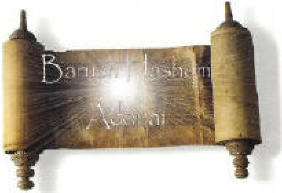INTERESTING PROPERTIES OF THE NUMBER 153

In John  21:11there is a reference where Peter drew the net from the Sea of Tiberias which held 153 fishes.
Some of the interesting properties of number 153 are:

If you add all the numbers from 1 to 17 together you get 153.

It is the smallest number which can be expressed as the sum of cubes of its digits:

153 = 13 + 53 + 33

It is equal to the sum of factorials of numbers from 1 to 5:

153 = 1! + 2! + 3! + 4! + 5!

The sum of digits of 153 is a perfect square:

1 + 5 + 3 = 9 = 32

The sum of aliquot divisors of 153 is also a perfect square:

1 + 3 + 9 + 17 + 51 = 81 = 92

Aliquot divisors of a number are all the divisors of that number excluding the number itself but including 1. It is seen that the sum of aliquot divisors of 153 is the square of the sum of the digits of 153.

On adding the number 153 to its reverse,504 is obtained, whose square is the smallest square which can be expressed as the product of two different numbers which are reverse of one another:

153 + 351 = 504

5042 = 288 x 882

It can be expressed as the sum of all integers from 1 to 17. In other words, 153 is the 17th triangular number. Since reverse of 153, i.e. 351 is also a triangular number, 153 can be termed as a reversible triangular number.

Number 153 is also a Harshad number (also called Niven number), i.e. it is divisible by the sum of its own digits:

153 / (1 + 5 + 3) = 17

Since reverse of 153, i.e. 351 is also a Harshad number (or Niven Number), 153 can be termed as a reversible Harshad number(or reversible Niven Number).